# Designing NOT Gate using Transistors

Published  December 9, 2019   0
M
Author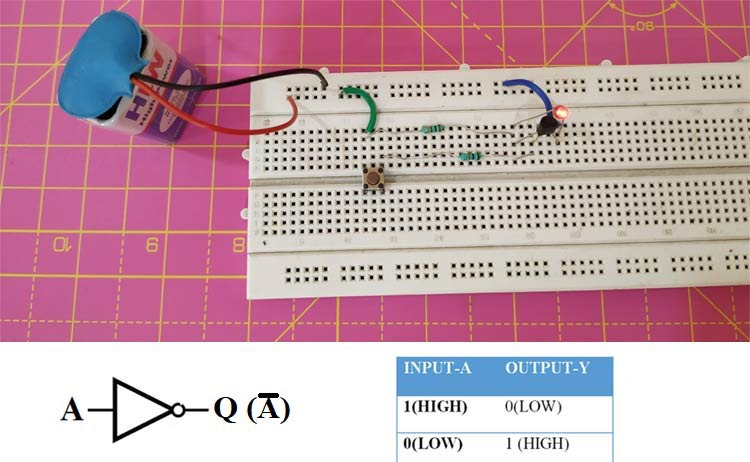An Integrated Circuit or IC is a combination of many small circuits in a small package that together performs a common task. For example, an Operational Amplifier or 555 Timer IC is built by a combination of many TransistorsFlip-FlopsLogic Gates and other combinational digital circuits. Similarly, a Flip-Flop can be built by using a combination of Logic Gates and the Logic Gates itself can be built by using a few Transistors.

In every IC’s, the basic block will be the logic gates whose outputs are either high (1) or low value (0). These logic gates will come under digital circuits. There are different types of logic gates, they are AND, OR, NOT, NAND, NOR gate, X-OR gate, and X-NOR gate. Among these, AND, OR, NOT are basic gates, while NOR and NAND gates are called universal gates. While each logic gate is available as an IC package ready to be used, it is also possible to build them using a simple article. We have already built an AND Gate using Transistor and an OR Gate using Transistor following that in this article we will build NOT gate using BJT Transistor. Before we begin let's understand the basics of NOT gate and transistors with their working.

### NOT Gate Basics and Working

NOT gate is the simplest gate when compared to the remaining digital logic gates. The NOT gate symbol is shown below, along with the NOT gate truth table. It has one input and one output.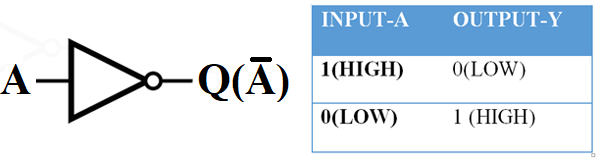The NOT Gate Boolean equation can be written as Y = , its output will be low when the input is high, and the output will be high when the input is low.

### Transistor – Basics, and Working

We are going to learn about transistors as we are going to build a NOT gate using BC547, which is an NPN transistor. A transistor is a back to back connection of a diode. A diode is a semiconductor device, which is doped with impurities to make it either a p-type or n-type depending on the types of impurities used in doping. When these diodes are connected in back to the back connection, they form a transistor. Depending on which both sides are connected, the transistors are of two types namely NPN Transistor and PNP Transistor.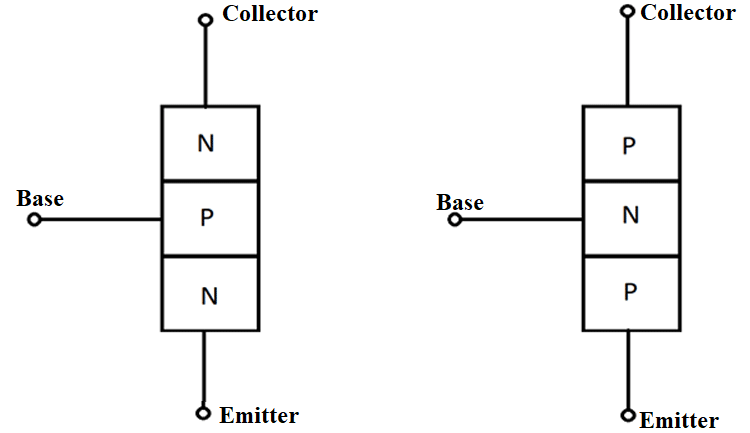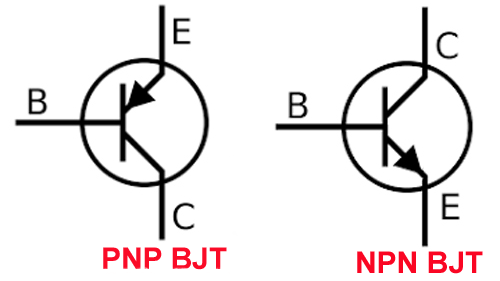The difference in circuit wise is that when connecting supply terminals, PNP transistor's emitter terminal is connected with the positive terminal, and for NPN transistor, the positive terminal is given to the collector terminal. From now on, the topic will be discussed based on only the NPN transistor.

Case 1: When base voltage is less than the emitter voltage, the flow of electrons from emitter to collector is blocked by the PN junction (this current is electric current which flows from negative terminal to positive terminal while convention current flows from positive terminal to negative terminal) as it is now acting in reverse bias.

Case 2: When the base voltage is greater than the emitter voltage (Vb > 0.6v), the junction gets reduced, and this allows the flow of current from the emitter terminal to the collector terminal. The transistor must work in a saturation region as they provide a low voltage drop in the saturation region.

### Circuit Diagram

The circuit for NOT gate using a transistor is given below. The circuit was designed and simulated using the Proteus software.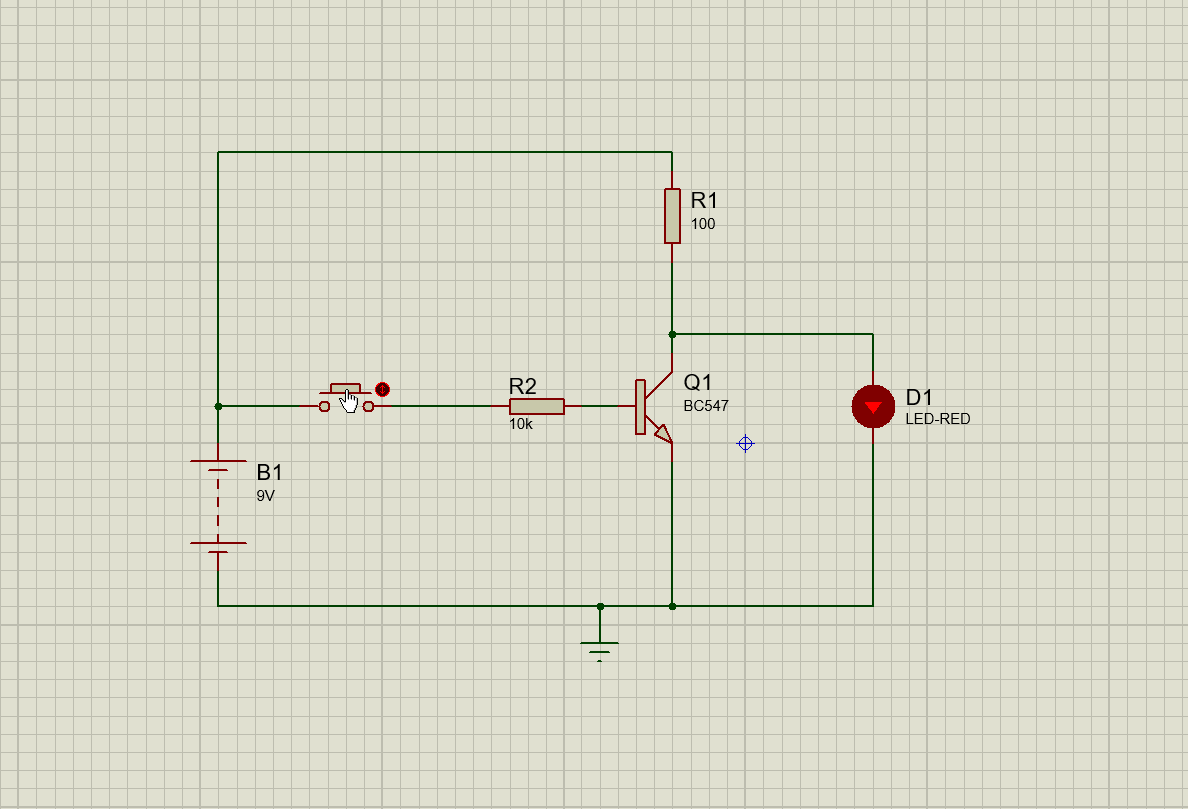I took supply voltage as 9V, and I want to send 9mA to led, so I used 100 ohms to limit the current. This same current has to flow in the transistor Ic = 9mA. The hfe of the transistor is 100, so Ib value should be 0.09mA. As the Ib is 0.09mA, the base resistor value should be 10k ohms.

The below fig shows the flow of current in both cases.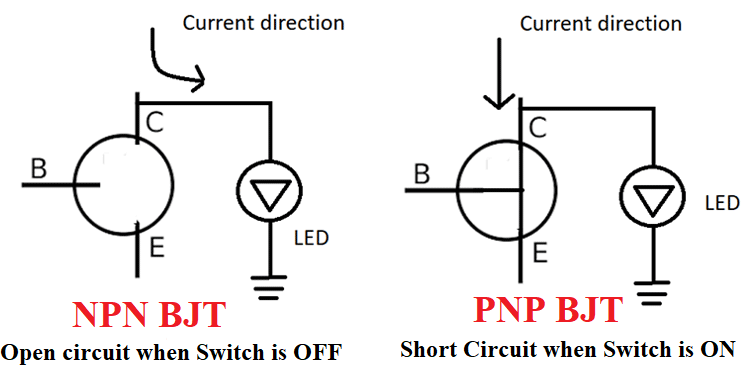Case 1:-

When the switch is in an off state, the current to the base is zero and transistor acts as open circuit due to these current flows in the LED direction and led starts glowing.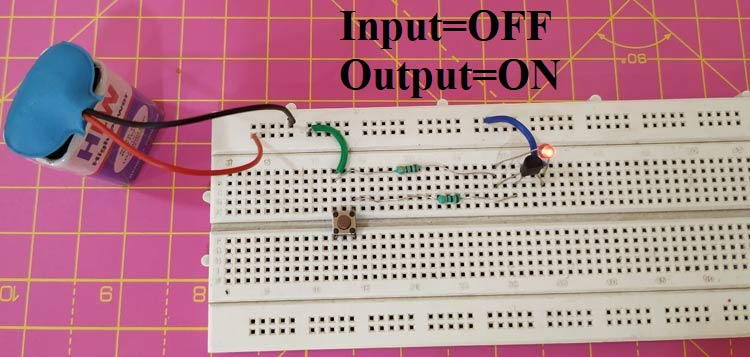Case 2:-

When the switch is in the ON state, the current to the base starts flowing, and this makes the transistor act as short circuit, and as the current choose the lowest resistance, which is now provided by the transistor will flow in that path and LED will be switched OFF.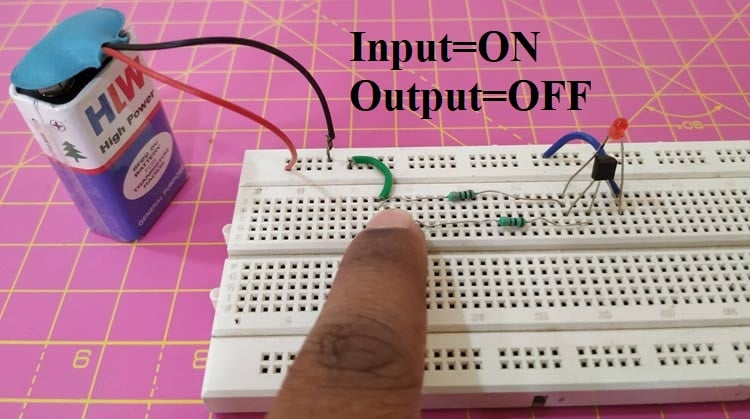Hence, both cases have the same inputs and outputs following the NOT gate truth table. Thus, we have built a NOT Logic gate using a Transistor. Hope you understood the tutorial and enjoyed learning something new. The complete working of the set-up can be found in the video below. If you have any questions leave them in the comment section below or use our forums for other technical questions.

Video